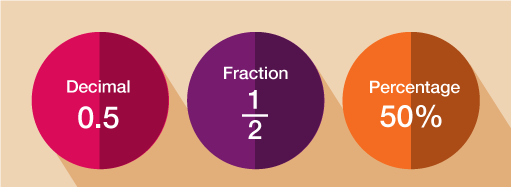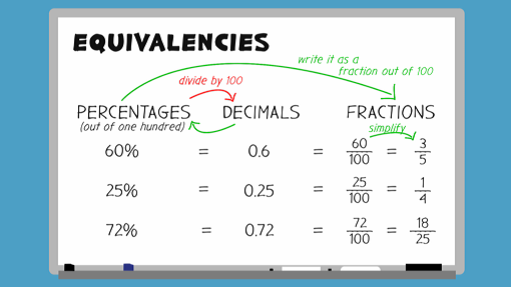Everyday maths 2

Start this free course now. Just create an account and sign in. Enrol and complete the course for a free statement of participation or digital badge if available.

Free course

# 6 Fractions, decimals and percentages

You have already worked with decimals in this course and many times throughout your life. Every time you calculate something to do with money, you are using decimal numbers. You have also learned how to round a number to a given number of decimal places.Figure 8 Equivalent decimals, fractions and percentages

Since fractions, decimals and percentages are all just different ways of representing the same thing, we can convert between them in order to compare. Take a look at the video below to see how to convert fractions, decimals and percentages.

Download this video clip.Video player: bltl_1_6_fractions_decimals_percentages.mp4Skip transcript

#### Transcript

Equivalent fractions, decimals, and percentages are all just different ways of representing the same thing, so you can convert between them to compare. First, let's look at turning percentages into decimals. Take the example of 60%. Remember that a percentage is out of 100. To turn a percentage into its equivalent decimal, you need to divide by 100. 60 divided by 100 = 0.6. So 0.6 is the equivalent decimal of 60%.

What about 25%? Can you work out the equivalent decimal of 25% before the answer is revealed? Remember that 'cent' means 100.

To turn 25% into its equivalent decimal, you need to divide 25 by 100, which = 0.25.

Now let's find the equivalent fraction for the decimals we've calculated. To do this, you need to first turn it into a percentage and write it as a fraction out of 100. Using our previous examples, 0.6 or 60%, can be written as 60 over 100. 0.25, or 25%, can be written as 25 out of 100.

However, these fractions are not written in their simplest form. Remember that to simplify fractions, you need to divide both parts by the same number and keep going until you can't find a number that you can divide both parts by. In their simplest form, 0.6 is written as the fraction 3/5. 0.25 is written as the fraction one quarter, or 1 over 4.

Now looking at the table, you can see how percentages, decimals and fractions relate to each other.

Let's try one more example. Can you work out the equivalent decimal and fraction of 72%? 72 divided by 100 gives the decimal 0.72. To find the equivalent fraction, you need to change it into a percentage and then write it as a fraction out of 100. Then simplify the fraction into its simplest form, 18 out of 25, 18/25.

End transcript

Interactive feature not available in single page view (see it in standard view).

## Activity 12: Matching fractions, decimals and percentages

Choose the correct fraction for each percentage and decimal.

Using the following two lists, match each numbered item with the correct letter.

• a.      62.5% = 0.625 =

• b.      40% = 0.4 =

• c.      50% = 0.5 =

• d.      35% = 0.35 =

The correct answers are:
• 1 = d
• 2 = b
• 3 = c
• 4 = a

Fractions and percentages deal with splitting numbers into a given number of equal portions, or parts. When dealing with the next topic, ratio, you will still be splitting quantities into a given number of parts, but when sharing in a ratio, you do not share evenly. This might sound a little complicated but you’ll have been doing it since you were a child.

## Summary

In this section you have:

• learned about the relationship between fractions, decimals and percentages and are now able to convert between the three.
FSM_2

### Take your learning further

Making the decision to study can be a big step, which is why you'll want a trusted University. The Open University has 50 years’ experience delivering flexible learning and 170,000 students are studying with us right now. Take a look at all Open University courses.

If you are new to University-level study, we offer two introductory routes to our qualifications. You could either choose to start with an Access module, or a module which allows you to count your previous learning towards an Open University qualification. Read our guide on Where to take your learning next for more information.

Not ready for formal University study? Then browse over 1000 free courses on OpenLearn and sign up to our newsletter to hear about new free courses as they are released.

Every year, thousands of students decide to study with The Open University. With over 120 qualifications, we’ve got the right course for you.

Request an Open University prospectus371